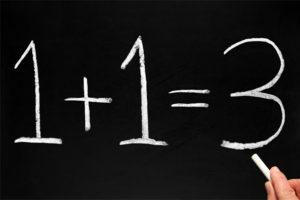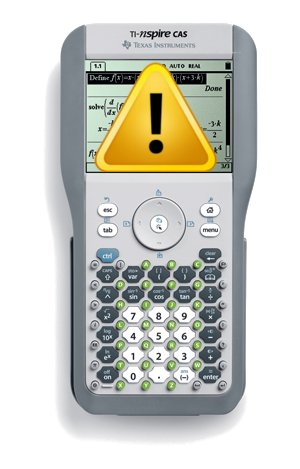Posted on

## Significant Figures and Scientific NotationFor many students, the concepts of significant figures and scientific notation are initially daunting and confusing. It is not unusual for a student to confuse the idea of rounding to a certain number of decimal places as being the same as rounding to a certain number of significant figures. Adding the concept of scientific notation into the mix can lead to the student being so uncertain as to how to write their answer for a given question.

Posted on

## Statistics in “APA Format”Mathematics is an ancient discipline, developed through many cultures and with many different ways of representing numbers and operations. Fortunately, there is a accepted convention that now governs how mathematics is represented around the world and it is through this convention that many mathematicians are able to exchange ideas independent of whether they speak the same (non-mathematical) language. It is not surprising, therefore, that statistics (a branch of mathematics) also has a convention on how statistics are represented.

## Statistical Conventions

In the statistics discipline we are typically dealing with one of two groups, the population or the sample. The population we are working with should be denoted by a capital Roman letter (e.g.$X$ ) and an individual from that population should be denoted with a lower case Roman letter (e.g.$x$ ). When we take a sample from the population, we are getting a set of$x$ ‘s out of our population$X$  (i.e each$x \in X$ )

Posted on

## Infallible Calculator?You may have seen the claim on the Internet that millions of people get the answer to the equation$6 \div 2\left(1 + 2\right)$  (or similar) wrong. You may have even read the explanations by some people about why the answer should be 9 or should be 1. Let me clear it up and explain why the answer is most definitely 1, but why the calculator (and others) give 9.

Firstly, the important thing to recognise is that this equation, like all others, is subject to the Order of Operations, which states that we must solve the contents of the brackets first. Thus our equation becomes$6 \div 2(3)$ .

Posted on

## Proof that 1 + 1 = 1. Not 2!?!Here is a quick demonstration of what can go wrong when you violate the rules of mathematics.

1. Let$a = 1$  and$b = 1$ .

Posted on

## The Secret to Success in MathematicsThere are so many people who struggle with mathematics and yet some people seem to have no trouble whatsoever. Is there something different in their DNA or some super advanced part of their brains, or do they simply know some secret trick that helps them in their understanding?

The answer is a little of the second, but mostly the last. Scientific research suggests that those who are good at mathematics do have some areas of their brain more active during mathematical activities, however, this increased brain activity could be a result of increased mathematical training. Much like how an athlete’s muscles improve with training, regular mathematical exercises help to improve the performance of those brain areas associated with mathematics. Again, like an athlete in training, there needs to be some underlying skills and a good coach. All of us possess the minimum underlying skills, but not all of us are fortunate enough to have a good coach at the critical time when we are ready to start serious mathematical training.